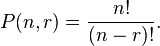My initial interest in permutations came from combinatorics.

In combinatorics, a permutation is usually understood to be a sequence containing each element from a finite set once, and only once. The concept of sequence is distinct from that of a set, in that the elements of a sequence appear in some order: the sequence has a first element (unless it is empty), a second element (unless its length is less than 2), and so on. In contrast, the elements in a set have no order; {1, 2, 3} and {3, 2, 1} are different ways to denote the same set.

However, there is also a traditional more general meaning of the term “permutation” used in combinatorics. In this more general sense, permutations are those sequences in which, as before, each element occurs at most once, but not all elements of the given set need to be used.

In this section only, the traditional definition from combinatorics is used: a permutation is an ordered sequence of elements selected from a given finite set, without repetitions, and not necessarily using all elements of the given set. For example, given the set of letters {C, E, G, I, N, R}, some permutations are ICE, RING, RICE, NICER, REIGN and CRINGE, but also RNCGI – the sequence need not spell out an existing word. ENGINE, on the other hand, is not a permutation, because it uses the elements E and N twice.

If n  denotes the size of the set – the number of elements available for selection – and only permutations are considered that use all n  elements, then the total number of possible permutations is equal to n!, where “!” is the factorial operator. This can be shown informally as follows. In constructing a permutation, there are n  possible choices for the first element of the sequence. Once it has been chosen, n − 1 elements are left, so for the second element there are only n − 1 possible choices. For the first two elements together, that gives us

n × (n − 1) possible permutations.

For selecting the third element, there are then n − 2 elements left, giving, for the first three elements together,

n × (n − 1) × (n − 2) possible permutations.

Continuing in this way until there are only 2 elements left, there are 2 choices, giving for the number of possible permutations consisting of n − 1 elements:

n × (n − 1) × (n − 2) × … × 2.

The last choice is now forced, as there is exactly one element left. In a formula, this is the number

n × (n − 1) × (n − 2) × … × 2 × 1

(which is the same as before because the factor 1 does not make a difference). This number is, by definition, the same as n!.

In general the number of permutations is denoted by,, or sometimes, where:

• n  is the number of elements available for selection, and
• r  is the number of elements to be selected (0 ≤ rn).

For the case where r = n  it has just been shown that P(n, r) = n!. The general case is given by the formula:This type of math came up recently when someone was helping me understand how to calculate the permutations in K4 if we only use 90 letters.  I won’t lie to you and say I understand it perfectly but I’m striving to understand it better.